# Force calculation: mv2/r, but here mv2r, why? [Proof of F = mv /R]

F calculation - mv2/r, but here mv2r, why?

This post was part of TyroCity discussion forum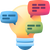Discuss User 2

From Newton’s second Law, it can be shown that F = ma, where F is the net force acting on a body, m is the mass of the body and a is the acceleration. So to find the centripetal force we must first find the centripetal acceleration for a body undergoing circular motion.

The following is from a previous answer of mine where I derived the centripetal acceleration:

A particle in circular motion follows the graph of x2+y2=r2, where r is the radius of the circular motion. This can be expressed in parametric equations with the time variable, allowing us to see where the particle would be at a specific point in time.

These are: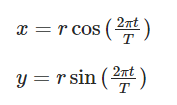Where T is the time period of the motion.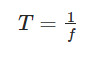Where f is the frequency and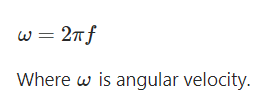Therefore the parametric equations can be re-written as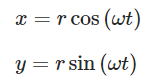Now we need to find the acceleration for the x and y components of position. We find these by double differentiating each position variable with respect to time.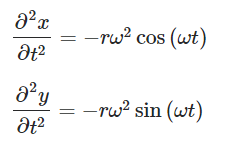Now we just need to find the resultant acceleration.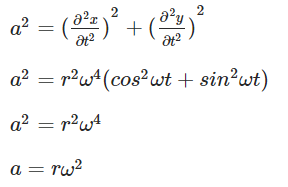Let’s now write this in a different form.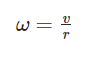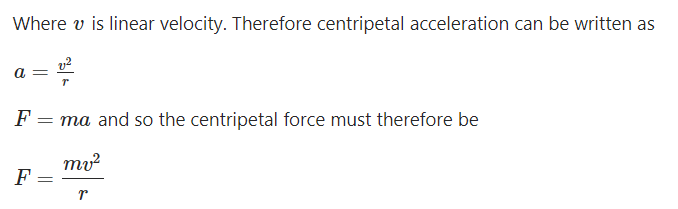This post was part of TyroCity discussion forum